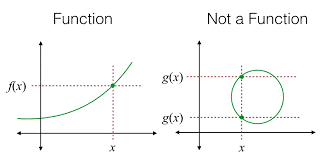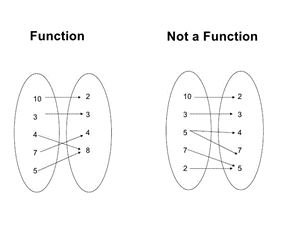Mathematics

# Relations and Functions

0

 1 Introduction 2 Relations and Functions 3 How to determine if a relation is a function? 4 Types of Functions 5 Types of Relations 6 Summary 7 FAQs 8 External References

15 December 2020

## Introduction

Relations and functions are one of the most critical topics of algebra. On most occasions, many people tend to confuse the meaning of these two terms.

A good fundamental base of these topics helps immensely to understand complex topics like calculus, algebra, etc., and even useful in other subjects like Physics, chemistry, and even economics.

The concept of a function was brought to light by mathematicians in the 17th century. In 1637, a mathematician and the first modern philosopher, Rene Descartes, talked about many mathematical relationships in his book Geometry, but the term “function” was officially first used by German mathematician Gottfried Wilhelm Leibniz after about fifty years.

He invented a notation y = x to denote a function, dy/dx to denote the derivative of a function. The notation y = f (x) was introduced by the Swiss mathematician Leonhard Euler in 1734.

Relations and Functions-PDF

In this blog, we will talk about Relations and functions, the differences between relation vs function, the situation when a relationship becomes a function, the types of both relations and functions, etc. Here is a downloadable PDF to explore more.

## Relations and Functions

A relation is just a collection of ordered pairs. The first number is called the input, and the second number is called the output. The set of input numbers is called the Domain of the Relation, and the set of output numbers is called the Range of Relation.

For example, {(-2, 3), {4, 5), (6, -5), (-2, 3)}, is a relation. It contains four ordered pairs. The first number can be called the x-coordinate, while the second is called the y-coordinate. For ordered pair (4,5), 4 is the x-coordinate, and 5 is the y-coordinate.

A function is also a relation but with one more extra rule. This additional rule says that each x-value should be associated with only one y-values and not more.

For example, y = x is a function because one x will have only one y value here.

## How to determine if a relation is a function

Now, let us talk about how to tell if something is a function or not and how to determine if a relation is a function. This is a confusing topic for many students, so please pay close attention to how to determine whether the relation is a function. Functions vs Relation is an important topic, and it strengthens your fundamental understanding of algebra and relations and functions fundamentally.

A function takes an input and produces a unique output while, on the other hand, a relation can produce different outputs for the same input. For example, $$y^2=x$$ , if you put x = 9, you will get two answers for y, which are 3 and -3. So $$y^2=x$$ is not a function.

Another example, $$y=x,$$, always has a unique y corresponding to each x. So $$y^2=x$$, it can be called a function. There is a test to determine whether the relation is a function. It is called the vertical test.

In the vertical test, we plot an x-y graph of the function, and then we move a vertical line from left to right( or vice-versa) on the graph. If the vertical line cuts the graph at more than one point at any instant, the given relationship is not a function. You can see it in the image below:Also, it can be explained with the mapping of x and y. If there is exactly one line originating from each x, then it is a function. If multiple lines are originating from one x, it is not a function.As another example,  take the relation {(-2, 3), {4, 5), (6, -5), (-2, 5)}, here we see that -2 is connected to two different y-values, 3 and 5. So it is not a function but just a relation. If we tweak the above Relation to {(-2, 3), {4, 5), (6, -5), (1, 5)}, here no x has two different y values, so it is a function.

Let’s go through a couple of examples and determine whether the relation is a function or not:

 Example 1

Determine if the relation {(-2, 3), (-1,3)} is a function.

Solution

Here in the relationship, we see that -2 is connected to 3, and -1 is also connected to 3. Recall the rule that we defined above. The given relation is a function because each input is connected to only one output. -2 and -1 each has only one output which is 3. If any of them had multiple outputs, the given relation would not be a function. A simple thumb rule is that the y-values can repeat but the x-values should not.

 Example 2

Determine if $$y^2=4x$$ is a function.

Solution

This one is a bit tricky. As we are not given some directly ordered pairs to look at, let us try possible values of x. If we put x=0 in the above equation, y comes out to be 0 as well. x=0 has only one y value, and the ordered pair is (0,0). So far, it looks like a function,

Let us try another value of x, say x=1. Replacing x in the given equation will give $$y^2=4$$, solving which y comes out to be either 2 or -2. The ordered pair will be (1,-2) and (1,2). So as we have two different y values for one x, the relation represented by the above equation is not a function.

## Types of Functions

There are many different types of functions that we encounter in mathematics. We will define them here:

1. One-One functions

Also called Injective functions, are the functions where each input is connected to a unique output. If x1 is connected to y1, then there will be no other input x in the domain which will be connected to y1.

2. Many-One function

It is the opposite of One-One functions. In this type, more than one input x’s can be connected to the same y.

3. Onto functions

Also called Surjective functions, they are the functions where each of the y that exist in the codomain is connected to x.

4. Into functions

5. They can contain some values in codomain which are not connected to the input set.

A function that is both one-one and onto is called Bijective functions. If we are told to find the inverse of a function, the function must be bijective otherwise it is not possible to find the inverse.

Some functions that are commonly encountered are :

1. Constant function

There is only one output value for all the input values of x. Their graph appears as a straight line parallel to the x-axis.

2. Polynomial functions

These functions look like the image below

$f(x)=a_nx^n+a_{n-1}x^{n-1}+\dots+a_0,$

The highest power of x is called the degree of the polynomial. Here, $$a_n,\ a_{n-1},................,\ a_1,\ a_0$$ are the coefficients of respective powers of x. The linear function and quadratic functions are special cases of polynomial functions.

3. Linear functions

These functions are just a linear combination of variables with coefficients. For example, y = 2x + 3 is a linear function. They appear as straight lines in the x-y axis graph.

4. Trigonometric functions

These functions are composed of various trigonometric ratios.

There are many other types of functions as well that we have not touched upon in this blog. Some are very complex that you will encounter later.

## Types of Relations

Let us define a set A. We can now form a relation R from A to A. Let A = {1,2} and now, let us look at different types of relations that can be:

1. Universal Relation

If all elements of A are related to every element of A, then it is a universal relation. For example,the universal relation R on A will be, {(1,1), (2,2), (1,2), (2,1)}.

2. Reflexive Relation

For every element ‘a’ in set A, if (a, a) exists in the relation R, then R is reflexive. For example, if (1,1) and (2,2) are both part of relation R, hence, R is reflexive.

3. Symmetric Relation

For every ordered pair (a,b) in R, if the pair (b, a) also exists in R, then R is symmetric. If (1,2) and (2,1) are both present in R, it is symmetric.

4. Transitive Relation

For every (a,b) and (b,c) that exist in R, if (a,c) is present then R is transitive.

5. Equivalence Relation

If a relation R is reflexive, symmetric, and transitive, then R is an equivalence relation.

6. Empty Relation

If none of the elements of set A are related to other elements of set A, then R is empty.

## Summary

In this blog, we learned about Relations and Functions, how to determine if a relation is a function, various types of functions, types of relations, etc.

These are some of the fundamental topics in algebra and calculus and a good knowledge of these helps a lot in complex mathematical topics. We highly encourage you to read more about this topic on your own.

You can also check out,

Cuemath, a student-friendly mathematics platform, conducts regular Online Live Classes for academics and skill-development, and their Mental Math App, on both iOS and Android, is a one-stop solution for kids to develop multiple skills.

Know more about the Cuemath fee here, Cuemath Fee

## What is a Relation?

A relation is just a collection of ordered pairs. For example, {(-2, 3), {4, 5), (6, -5), (-2, 3)}, is a relation. The first number is called the input and the second number is called the output.

## What is a Function?

A function is also a relation but with one more extra rule. This extra rule says that each x-value should be associated with only one y-values and not more

## How to determine if a relation is a function?

A function takes an input and produces a unique output while, on the other hand, a relation can produce different outputs for the same input. If you know the graph of the relation, you can determine whether a relation is a function through the Vertical test.